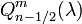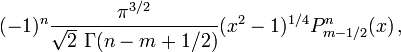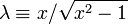User:Tohline/Math/EQ Toroidal02$~Q_{n-1 / 2}^m (\lambda)$$~=$$~(-1)^n \frac{\pi^{3/2}}{\sqrt{2}~ \Gamma(n-m+1 / 2)} (x^2-1)^{1 / 4} P_{m-1 / 2}^n(x) \, ,$ Gil, Segura, & Temme (2000):  eq. (8)
 where:$~\lambda \equiv x/\sqrt{x^2-1}$